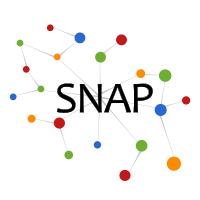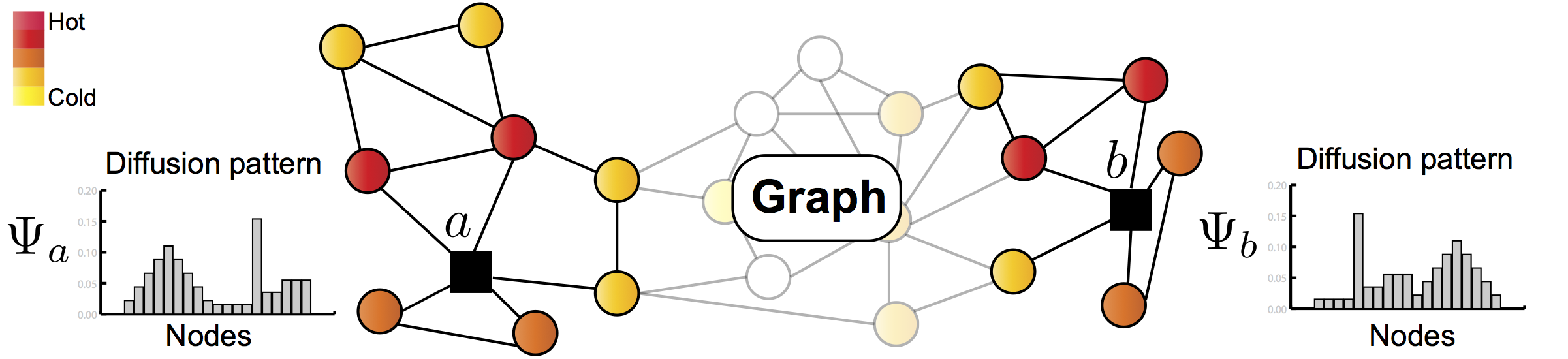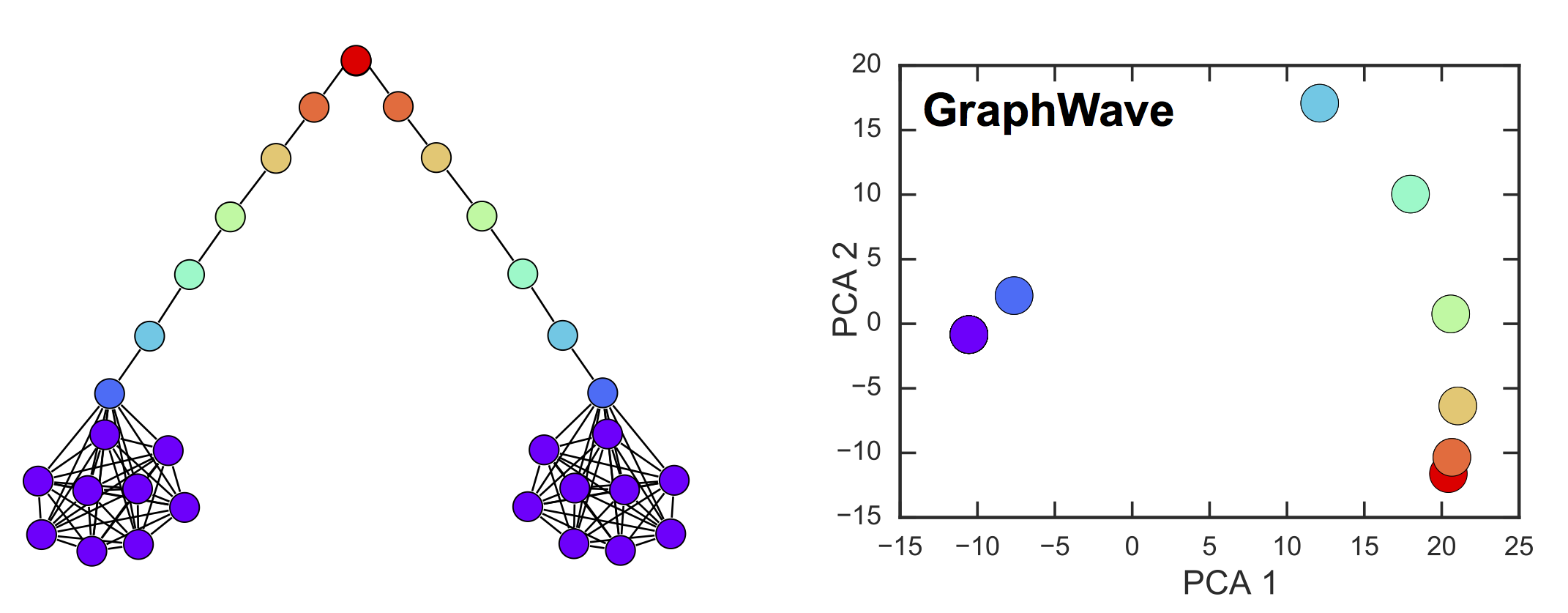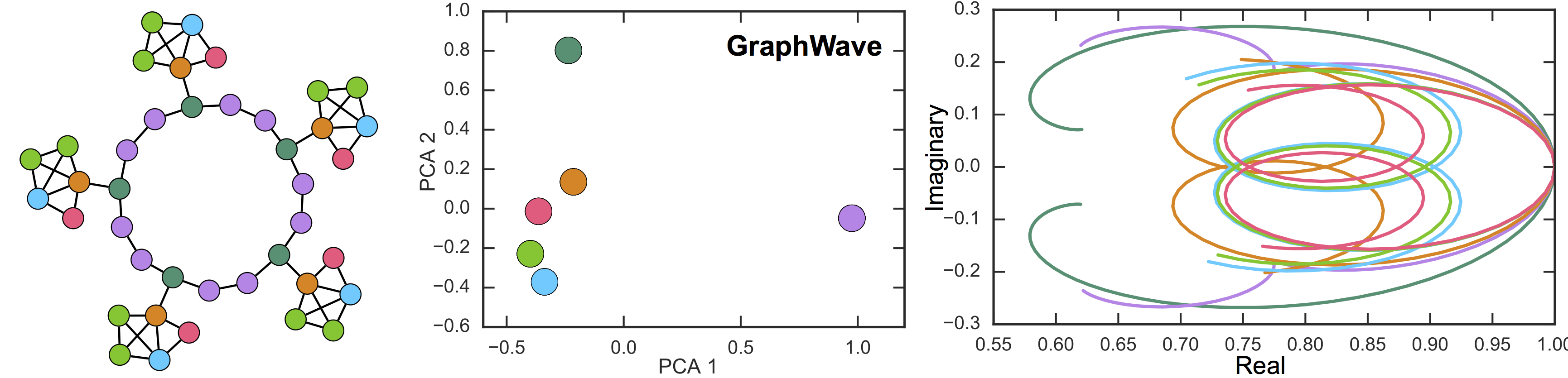# GraphWave: Learning Structural Node Embeddings

GraphWave is a scalable unsupervised method for learning node embeddings based on structural similarity in networks. GraphWave develops a novel use of spectral graph wavelets by treating the wavelets as probability distributions and characterizing the distributions using empirical characteristic functions.

Nodes residing in different parts of a graph can have similar structural roles within their local network topology. The identification of such roles provides key insight into the organization of networks and can also be used to inform machine learning on graphs. However, learning structural representations of nodes is a challenging unsupervised-learning task, which typically involves manually specifying and tailoring topological features for each node.

GraphWave is a method that represents each node's local network neighborhood via a low-dimensional embedding by leveraging spectral graph wavelet diffusion patterns.

GraphWave provides theoretical guarantees; nodes with similar local network neighborhoods will have similar GraphWave embeddings even though these nodes may reside in very different parts of the network. GraphWave scales linearly with the number of edges and does not require any hand-tailoring of nodes' topological features.

Learning Structural Node Embeddings via Diffusion Wavelets.
Claire Donnat, Marinka Zitnik, David Hallac, and Jure Leskovec.
KDD 2018. [Video]

@inproceedings{donnat2018, author={Donnat, Claire and Zitnik, Marinka and Hallac, David and Leskovec, Jure}, title = {Learning Structural Node Embeddings via Diffusion Wavelets}, year = {2018}, booktitle = {International ACM Conference on Knowledge Discovery and Data Mining (KDD)}, volume = {24} }

## GraphWave Approach

GraphWave learns a structural embedding for each node based on the diffusion of a spectral graph wavelet centered at that node. Intuitively, each node propagates a unit of energy over the graph and characterizes its neighboring topology based on the response of the network to this probe.

GraphWave uses a novel way of treating the wavelets as probability distributions over the graph. This way the structural information is contained in how the diffusion spreads over the network rather than where it spreads. In order to provide vector-valued signatures which can then be used as input to any machine learning algorithm, GraphWave embeds these wavelet distributions using the empirical characteristic function.In the figure above, nodes a and b have similar local structural roles even though they are distant in the graph. While raw spectral graph wavelet signatures/coefficients Ψ of a and b might be very different, GraphWave treats them as probability distributions and can thus automatically learn that the coefficient distributions are indeed similar. Graphwave leverages these new insights to learn a structural embedding for node a (b) based on the diffusion of a spectral graph wavelet centered at node a (b).

## Barbell Graph

In this example, we consider a barbell graph consisting of two dense cliques connected by a long chain. We apply GraphWave to the barbell graph and plot a 2D PCA of the learned structural signatures.

As can be seen in the figure below, the graph has 8 distinct classes of structurally equivalent nodes as indicated by color (left). 2D PCA projection of structural signatures (right) contain the same number of points as there are nodes in the barbell graph. This is because identical signatures have identical projections, resulting in overlapping points.GraphWave correctly learns identical representations for structurally equivalent nodes, providing empirical evidence for GraphWave's theoretical guarantees. This can be seen by structurally equivalent nodes in the figure (nodes of the same color) having identical projections in the PCA plot. In particular, GraphWave correctly groups the clique nodes (purple) together.

GraphWave also correctly differentiates between nodes connecting the two dense cliques in the barbell graph. It represents those nodes in a gradient-like pattern that captures the spectrum of structural roles of those nodes (right).

## A Cycle Graph with Attached House Shapes

In this example, we consider a graph where "house" shapes are placed regularly along a cycle graph. As before, we use GraphWave to learn structural signatures for nodes in the graph and then use ground-truth information about structural roles to evaluate GraphWave's performance.

The graph is visualized in the figure below (left), together with the 2D PCA projection of GraphWave's structural signatures (middle). We observe that the representation of structurally equivalent nodes overlap, and GraphWave perfectly recovers the 6 different node types.We also visualize the resulting characteristic functions for the distribution of wavelet coefficients (right). In the figure, different shapes of characteristic functions capture different structural roles. We note the visual proximity between the roles of the blue, light green and red nodes that these curves carry, as well as their clear difference with the core dark green and purple nodes.

## Code

A Python implementation of GraphWave is available on GitHub.